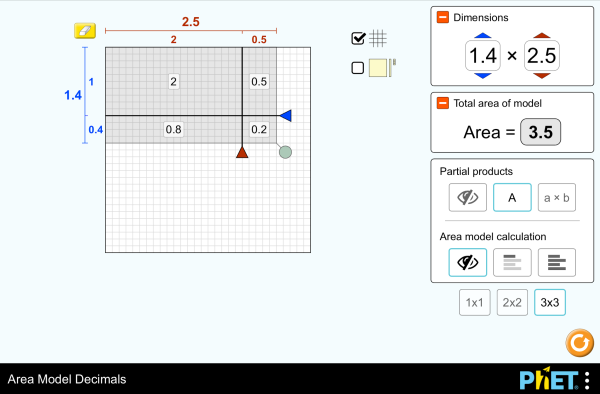# Area Model Decimals

Táto simulácia nebola preložená do slovenčiny. Zatiaľ použite anglickú verziu.StiahnuťKód na vloženie zatvoriť Vložiť bežiacu kópiu tejto simulácie Použite tento HTML kód pre vloženie tejto simulácie na svoju web stránku. Môžete taktiež zmeniť výšku a šírku vloženej simulácie zmenením atribútov "width" a "height" v HTML kóde. Vložiť obrázok, ktorý po kliknutí spustí simuláciu.
Kliknite pre spustenie
Použite tento HTML kód pre zobrazenie obrazovky simulácie s nápisom "Kliknite pre spustenie" Násobenie Decimals Area Model PhET podporujea pedagógovia ako ste Vy.

### Témy

• Násobenie
• Decimals
• Area Model
• Factors
• Partial Products

### Popis

Build rectangles of various sizes and relate multiplication to area. Partition a rectangle into smaller areas and discover new strategies for multiplying decimals!

### Ukážkové ciele vzdelávania

• Recognize that area represents the product of two numbers and is additive.
• Develop and justify a strategy that uses the area model to simplify a decimal multiplication problem.
• Devise a strategy to determine the product of two rational numbers (or an integer and a rational number) by representing the product as an area or the sum of areas.
• Use an area model to understand why multiplying a given number by a decimal less than 1 results in a product smaller than the given number.
• Interpret the product of two rational numbers using base-ten tiles

### Standards Alignment

#### Common Core - Math

5.NF.B.4
Apply and extend previous understandings of multiplication to multiply a fraction or whole number by a fraction.
5.NF.B.4a
Interpret the product (a/b) × q as a parts of a partition of q into b equal parts; equivalently, as the result of a sequence of operations a × q ÷ b. For example, use a visual fraction model to show (2/3) × 4 = 8/3, and create a story context for this equation. Do the same with (2/3) × (4/5) = 8/15. (In general, (a/b) × (c/d) = ac/bd.)
5.NF.B.4b
Find the area of a rectangle with fractional side lengths by tiling it with unit squares of the appropriate unit fraction side lengths, and show that the area is the same as would be found by multiplying the side lengths. Multiply fractional side lengths to find areas of rectangles, and represent fraction products as rectangular areas.
5.NF.B.5
Interpret multiplication as scaling (resizing), by:
5.NF.B.5a
Comparing the size of a product to the size of one factor on the basis of the size of the other factor, without performing the indicated multiplication.
5.NF.B.5b
Explaining why multiplying a given number by a fraction greater than 1 results in a product greater than the given number (recognizing multiplication by whole numbers greater than 1 as a familiar case); explaining why multiplying a given number by a fraction less than 1 results in a product smaller than the given number; and relating the principle of fraction equivalence a/b = (n × a)/(n × b) to the effect of multiplying a/b by 1.
Verzia 1.2.1

### Teacher-Submitted Activities

Games Remote Lesson ideasTrish Loeblein VŠ-zač

ZŠ-II
K-5

Remote
Chémia
Fyzika
Matematika
Area Model Decimals - Grade 6Maura Dudley ZŠ-II Guided Matematika
Middle School Math Sim AlignmentAmanda McGarry ZŠ-II Iné Matematika
PRIMARIA: Alineación con programas de la SEP México (2011 y 2017)Diana López K-5
ZŠ-II

Disk
Lab
Guided
Demo
Chémia
Matematika
Astronómia
Fyzika
Albánčina All shqipModeli i Syprinës: Decimal
Arabčina All العربيةنموذج مساحة الأعداد العشرية
Baskičtina All EuskaraAzalera-modeloa hamartarrekin
Bosnian All BosanskiMatematički model površina - Decimalni brojevi
Čínština (Tradičná) All 中文 (台灣)Area Model Decimals_面積模型與小數
Čínština (Zjednodušená) All 中文 (中国)面积模型：小数
Dánčina All DanskArealmodel, decimaler
Fínčina All suomiPinta-alamalli desimaaleilla
Francúzština All françaisModélisation par les aires avec décimales
Gréčtina All ΕλληνικάΥπολογισμός εμβαδού - Δεκαδικοί
Gujarati All Gujaratiક્ષેત્રફળનું મોડેલ - દશાંશ
Hindi All हिंदीनमूने का क्षेत्रफल - दाशमिक
Holandčina All NederlandsOppervlakte model decimalen
Japončina All 日本語面積と式の展開（小数）
Kazakh All KazakhАуданды ондық бөлшектермен есептеу
Kórejčina All 한국어면적 모형: 소수
Latvian All LatviešuLaukuma modeļa decimāldaļskaitļi
Maďarčina All magyarTerületmodell: tizedesjegyek
Marathi All मराठीअपूर्णांकातील क्षेत्रफळ
Mongolian All Монгол (Монгол)Талбайн Загвар Бутархайгаар
Nemčina All DeutschFlächenmodell Dezimalzahlen
Perzština All فارسیمدل مساحت اعشاری ها
Poľština All polskiModel pola - ułamki dziesiętne
Portugalčina (Brazília) All português (Brasil)Modelo de Área: Decimais
Ruština All русскийВычисление площади с десятичными дробями
Sinhalese All Sinhaleseවර්ගඑල ආකෘති දශම
Srbčina All СрпскиМатематика преко површина-Децимални бројеви
Španielčina All españolModelo de Áreas: Decimales
Španielčina (Mexico) All español (México)Modelo de Áreas: Decimales
Španielčina (Peru) All español (Perú)Modelo de área con decimales
Švédčina All svenskaAreamodellen och decimaltal
Taliančina All italianoRappresentazione grafica del prodotto di numeri decimali
Tamil All Tamilமாதிரிகளின் தசமப் பரப்பளவு
Turečtina All TürkçeAlan Modelinde Çarpma İşlemi (Ondalık)
Ukrajinčina All українськаПлощинна модель десяткових дробів
Vietnamčina All Tiếng ViệtSố thập phân với mô hình diện tích

HTML5 sims can run on iPads and Chromebooks, as well as PC, Mac, and Linux systems.

iOS 12+ Safari

Android:
Not officially supported. If you are using the HTML5 sims on Android, we recommend using the latest version of Google Chrome.

Chromebook:
The HTML5 and Flash PhET sims are supported on all Chromebooks.
Chromebook compatible sims

Windows Systems:

Macintosh Systems:

Linux Systems:

Autorský tím Iné knižnice Poďakovanie
• Jonathan Olson (developer)
• Karina Hensberry
• Susan Miller
• Ariel Paul
• Kathy Perkins
• almond-0.2.9.js
• base64-js-1.2.0.js
• FileSaver-b8054a2.js
• font-awesome-4.5.0
• game-up-camera-1.0.0.js
• he-1.1.1.js
• himalaya-0.2.7.js
• jama-1.0.2
• jquery-2.1.0.js
• lodash-4.17.4.js
• pegjs-0.7.0.js
• seedrandom-2.4.2.js
• text-2.0.12.js
• TextEncoderLite-3c9f6f0.js